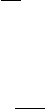1Newtonian dynamics of particles

IA Dynamics and Relativity1 Newtonian dynamics of particles
Newton’s equations describe the motion of a (point) particle.
Definition
(Particle)
.
A particle is an object of insignificant size, hence it can
be regarded as a point. It has a mass m > 0, and an electric charge q.
Its position at time
t
is described by its position vector,
r
(
t
) or
x
(
t
) with
respect to an origin O.
Depending on context, different things can be considered as particles. We
could consider an electron to be a point particle, even though it is more accurately
described by the laws of quantum mechanics than those of Newtonian mechanics.
If we are studying the orbit of planets, we can consider the Sun and the Earth
to be particles.
An important property of a particle is that it has no internal structure. It can
be completely described by its position, momentum, mass and electric charge.
For example, if we model the Earth as a particle, we will have to ignore its own
rotation, temperature etc.
If we want to actually describe a rotating object, we usually consider it as a
collection of point particles connected together, and apply Newton’s law to the
individual particles.
As mentioned above, the position of a particle is described by a position
vector. This requires us to pick an origin of the coordinate system, as well as an
orientation of the axes. Each choice is known as a frame of reference.
Definition
(Frame of reference)
.
A frame of reference is a choice of coordinate
axes for r.
We don’t impose many restrictions on the choice of coordinate axes. They
can be fixed, moving, rotating, or even accelerating.
Using the position vector
r
, we can define various interesting quantities which
describe the particle.
Definition (Velocity). The velocity of the particle is
v =
˙
r =
dr
dt
.
Definition (Acceleration). The acceleration of the particle is
a =
˙
v =
¨
r =
d
2
r
dt
2
.
Definition (Momentum). The momentum of a particle is
p = mv = m
˙
r.
m
is the inertial mass of the particle, and measures its reluctance to accelerate,
as described by Newton’s second law.